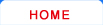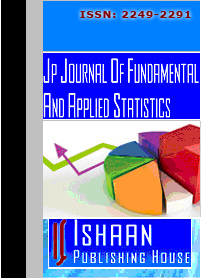Journal HomeEditorial BoardGuidelines for AuthorsSubscriptionContentOnline SubmissionJP Journal of Fundamental and Applied Statistics Editorial Board
 Abayomi, Kobi Department of Statistics Columbia University New York, NY 10027 U. S. A. [Statistical dependence, Imputation, Environmental economics, Econometrics, Measures of inequality] Akdeniz, Fikri Department of Statistics Çukurova University Adana 01330 Turkey [Linear models, Generalized inverses, Ridge regression, Liu-type estimators, Difference-based estimator, Jackknife estimator, Mixture distributions, Pre-test estimators, Stein-rule estimators, SUR models, Entropy estimators, Semiparametric regression models] Arslan, Olcay Department of Statistics Faculty of Science Ankara University 06100 TandoganAnkara, Turkey [Multivariate analysis, Regression analysis, Robust estimation, Distribution theory, Statistical computation, EM, iteratively reweighting algorithms] Berg, Arthur Department of Statistics Penn State University Gainesville, FL 32611 U. S. A. [Statistical genetics, Time series analysis, Nonparametrics] Cheng, Tsung-Chi Department of Statistics National Chengchi University 64 ZhihNan Road, Section 2 Taipei 11605 Taiwan [Detection of outliers, Robust estimation, Categorical data analysis, Statistical quality control] Cruyff, Maarten J. L. F. Department of Methodology and Statistics Utrecht University P. O. Box 80.140 3508 TC Utrecht The Netherlands [Randomized response, Population size estimation] Dolati, Ali Department of Statistics Faculty of Science Yazd University Yazd 89195–741 Iran [Multivariate distributions and theory of copulas, Dependence concepts and orderings, Stochastic orders] Fackle-Fornius, Ellinor Department of Statistics Stockholm University Stockholm Sweden [Optimal design of experiments, Minimax designs, Sequential designs, Generalized linear models] Ghosh, Dilip K. UGC BSR Faculty Fellow Retired Professor and Head Department of Statistics Saurashtra University Rajkot 360005, Gujarat India [Design of experiments, Optimal designs, Operations research, Bio-statistics, Estimation theory, Matrix algebra, Econometrics, Linear model] González-Farías, Graciela Department of Probability and Statistics Centro de Investigación en Matemáticas Callejón de Jalisco s/n Mineral de Valenciana 36240 Guanajuato, Guanajuato Mexico Hoque, Zahirul Department of Statistics UAE University P. O. Box 17555, Al Ain UAE [Improved estimation in linear models, Measurement error, Experimental design] Jiang, Jiancheng Department of Mathematics and Statistics University of North Carolina at Charlotte Charlotte, NC 28223 U. S. A. [Nonparametric smoothing, Survival analysis, Model selection, Robust Estimation, among others] Khaledi, Baha-Eldin Department of Statistics Razi University Kermanshah Iran Koyuncu, Nursel Department of Statistics Hacettepe University Beytepe, Ankara Turkey [Sampling, Ratio estimator, Repeated measurement, Ordinal response data] Ray, Surajit Department of Mathematics and Statistics Boston University 111 Cummington Street Boston, MA 02215 U. S. A. [Model selection, The theory and geometry of mixture models and functional data analysis, The dimension of data vectors and in the number of vector, multivariate mixtures, structural equations models, high-dimensional clustering and functional clustering, immunology, modeling of climate ecosystem dynamics and medical image segmentation] Xu, Liwen Department of Statistics College of Sciences North China University of Technology Beijing 100144 P. R. China [Parametric inference (62F03 Hypothesis testing, 62F10 Point estimation), Linear inference, Regression (62J05 Linear regression, 62J10 Analysis of variance and covariance), Multivariate analysis (62H12 Estimation, 62H15 hypothesis testing), The area of panel data analysis (or Longitudinal data analysis, Generalized p-value, Generalized confidence interval, Linear mixed effects model)] Watier, Francois Department of Mathematics Universite du Quebec a Montreal Montreal Canada [Stochastic control, Stochastic differential equations, Applied probability, Mathematical finance] Wang, Bing Xing Department of Statistics Zhejiang Gongshang University Hangzhou P.R. China [Censored data analysis, Accelerated life test data analysis] Woo, Jungsoo Department of Statistics Yeungnam University Gyongsan South Korea [Jaccknife and Bootstrap, Reliability, Outliers, Stochastic ordering, Probability distributions]
 © Ishaan Publishing House Best view with Mozilla Firefox 3.5.x, IE 6+ , Opera version at 1024x768 pixels resolution. ...